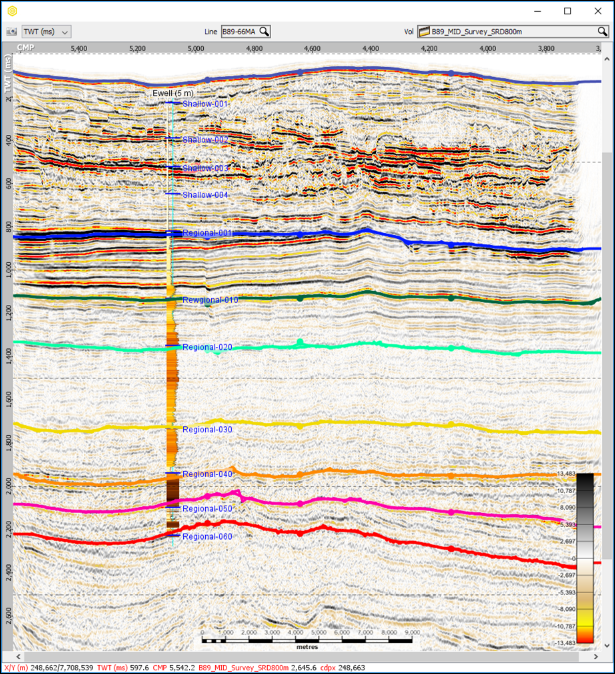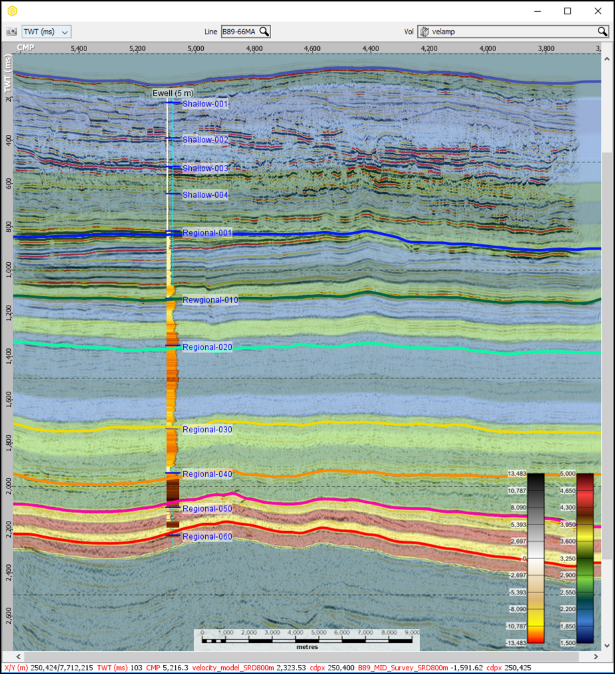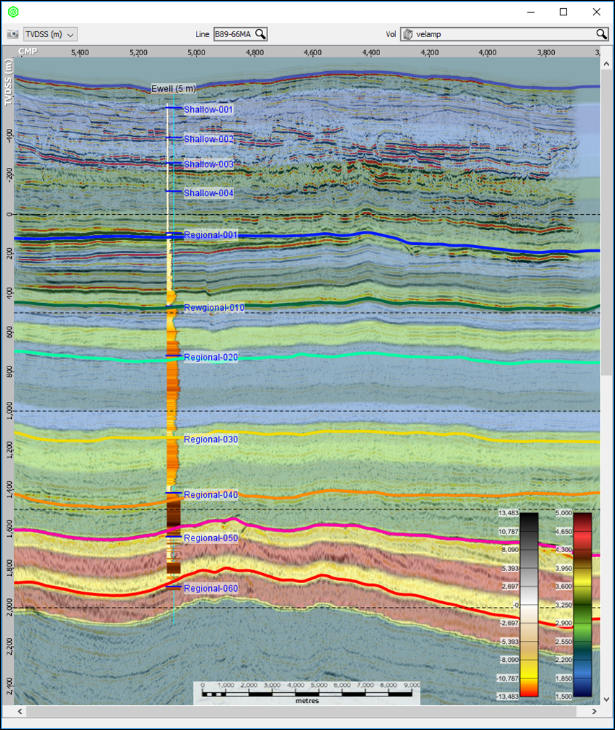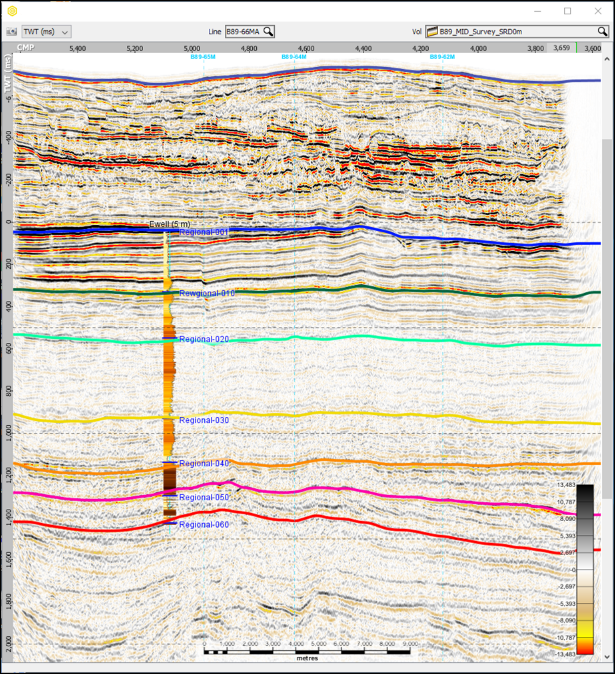# Seismic Reference Datums

Marine seismic is usually referenced to Mean Sea Level (MSL) which is a global datum for measuring height. In a marine project, zero elevation (the mean sea level) will have a time of 0 ms.

Because elevation on land is variable, land seismic is often referenced to a Seismic Reference Datum (SRD). The SRD is a plane that sits above the highest elevation in the data. In a land project with a seismic reference datum set, the SRD will have a time of 0 ms. All time data, e.g. seismic and velocities, will start at this datum.

The seismic reference datum will be above the elevation of most of the land surface. For seismic, values in the is gap will be empty. In velocity models, a replacement velocity fills the data gap between the surface and the datum. It’s an important value to note when manipulating volumes with different datums.In a project, all wells and traces are referenced to MSL enabling objects to be compared to each other.

• Seismic is Measured from MSL or SRD
• Well data is most commonly referenced to KB, DF or RT.
• Checkshots are most commonly referenced to SRD, MSL and sometimes KB
• GL or ML(WB) can also be used but are not as common.

In Insight, the default seismic reference datum is 0m. With marine data you do not need to change this value. There are two ways to configure a project with land data referencing a non zero SRD.

## Method 1 - Set a seismic reference datum for the project

In Configure project, set the SRD to match the seismic survey SRD.

In this example 800 m = 0 ms. The replacement velocity is 2000 m/s.The well has its own datum set at 613 m above MSL.With the seismic reference datum configured as 800m:

In TWT

• The line at time zero is the SRD, 800 m above MSL
• Unconverted TWT traces begin at time zero, all the samples are after time zero.
• The entire well converted to TWT is visible.
• The wells and seismic tie.
• Unconverted TWT-Interval Velocity data (inset image) starts from time zero.In TVDSS

• The line at depth zero is the MSL.
• TWT traces converted to TVDSS start at the SRD, 800 m above MSL.
• The unconverted depth well is fully visible.
• The wells and seismic tie.
• The TWT-Interval Velocity data (inset image) converted to TVDSS starts from time zero, 800 m above MSL

This is ideal. All the data is displayed in both TWT and TVDSS, and the velocity model extends to the seismic reference datum. Time-depth conversion using the model covers the entire vertical domain.

## Method 2 - Use Mean Sea Level (0m) as the seismic reference datum

The trace data was originally processed using a SRD of 800m. The time traces have been shifted using replacement velocity of 2000 m/s from the original SRD of 800 m to a new 0 m MSL datum.

In Configure project, set the SRD to 0m (MSL).

In this example: 0 m = 0 ms.The well has its own datum set at 613 m above MSL.With the seismic reference datum set to MSL (0 m):

In TWT

• The line at time zero is the MSL
• Unconverted TWT Traces begin at time -800 ms, the time shift calculated from the original SRD using a replacement velocity of 2000 m/s.
• The entire TWT trace is visible above and below time zero (MSL).
• The well converted to TWT is only visible below 0 ms. “Negative time” values are not displayed.
• Wells and seismic tie for the visible portion.
• Unconverted TWT-Interval Velocity data starts at time zero. “Negative time” values are not displayed.In TVDSS

• The line at depth zero is the MSL.
• The TWT traces converted to TVDSS are only visible below 0 m. “Negative Depth” values are not displayed.
• The entire unconverted Depth well is visible
• The wells and seismic tie for the visible portion
• The TWT Interval Velocity data (inset image) converted to TVDSS is only visible below 0 m. “Negative Depth” values are not displayed.

This method truncates well data at MSL when displayed in TWT, and seismic time data at MSL when displayed in TVDSS. The velocity model is modelled from below MSL, with only the constant replacement velocity available to use above MSL.

For a single seismic reference datum this isn't the best method, but this method becomes useful when using multiple surveys with multiple seismic reference datums. After defining a common seismic reference datum, each survey is shifted to align with each other.